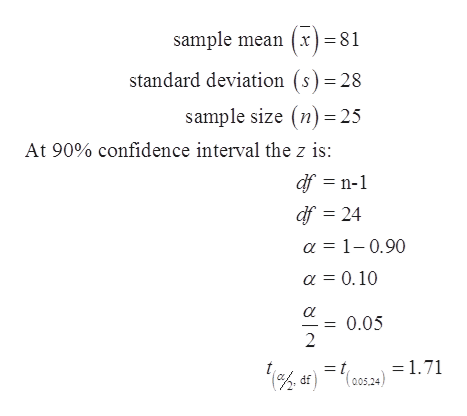# If upper overbar X=81​, Upper S=28​, and n=25​,and assuming that the population is normally​ distributed, construct a 90%confidence interval estimate of the population​ mean,μ. [   ]≤ μ≤[  ]​(Round to two decimal places as​ needed.)

Question
If upper overbar X=81​, Upper S=28​, and n=25​,and assuming that the population is normally​ distributed, construct a 90%confidence interval estimate of the population​ mean,μ.

[   ]≤ μ≤[  ]
​(Round to two decimal places as​ needed.)
check_circle

Step 1

From the provided information, populati...help_outlineImage Transcriptionclosesample mean (x) =81 standard deviation (s) 28 sample size (n)25 At 90% confidence interval the z is: df n-1 df 24 a 1-0.90 a = 0.10 0.05 = 1.71 fullscreen

### Want to see the full answer?

See Solution

#### Want to see this answer and more?

Solutions are written by subject experts who are available 24/7. Questions are typically answered within 1 hour.*

See Solution
*Response times may vary by subject and question.
Tagged in

### Other